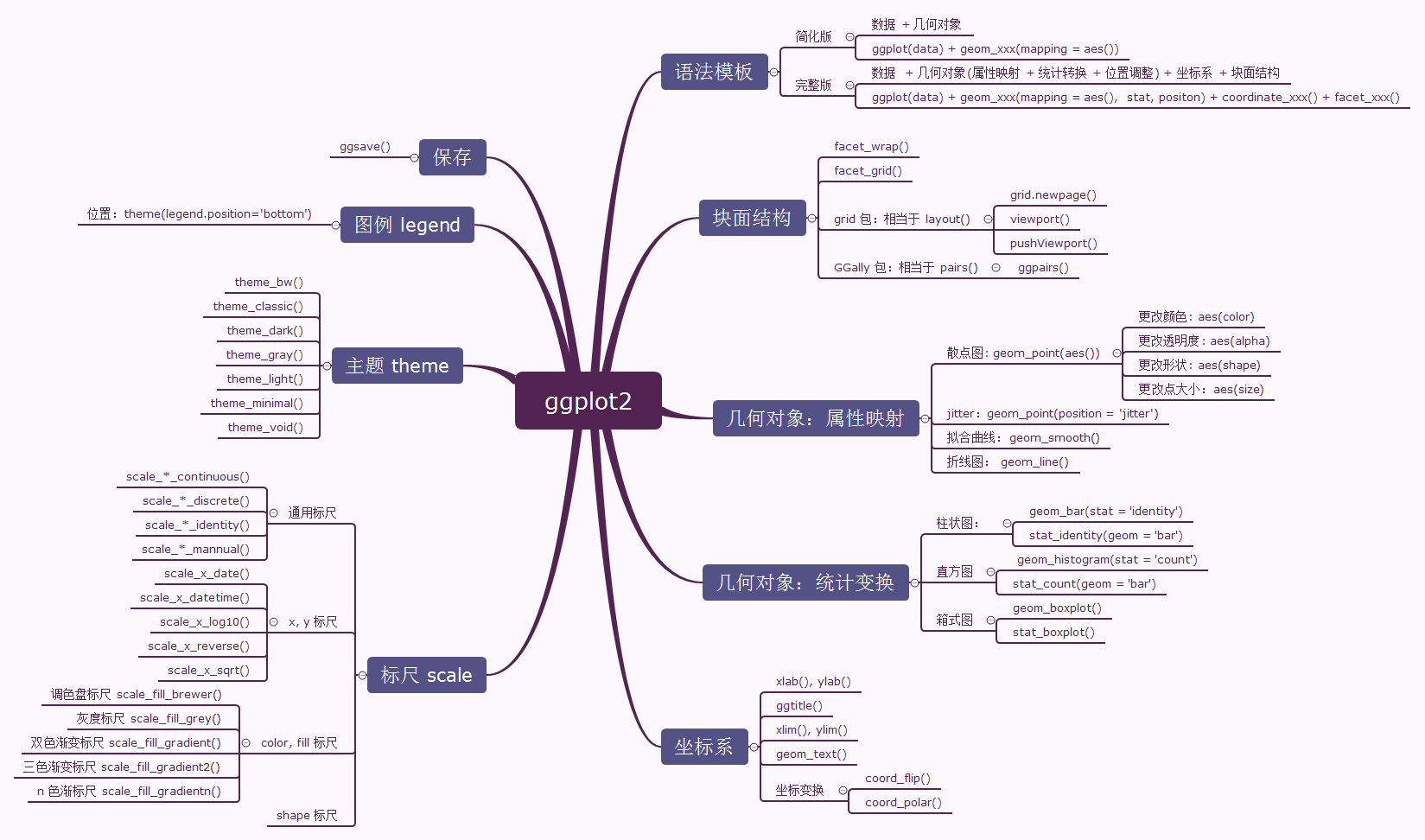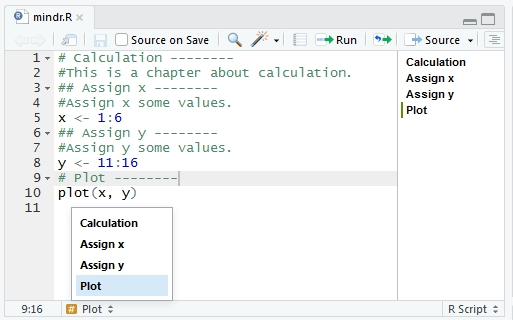# 用 markdown 的逻辑写 R 脚本注释

— 《学 R》第一章 “初见”

## R 脚本到思维导图## .R 变成 .Rmd

.R .Rmd
# + 空格” 开头的注释行 保留不变，自动成为章节标题
# + 非空格字符”开头的注释行 去掉开头的#，成为正文文字

# Calculation --------
#This is a chapter about calculation.
## Assign x --------
#Assign x some values.
x <- 1:6
## Assign y --------
#Assign y some values.
y <- 11:16
# Plot --------
plot(x, y)mindr::r2md() 转换得到的 .Rmd 是这样的：

# Calculation
This is a chapter about calculation.
## Assign x
Assign x some values.
​{r}
x <- 1:6
​
## Assign y
Assign y some values.
​{r}
y <- 11:16
​
# Plot
​{r}
plot(x, y)
​## .Rmd 变成 .R

#' # Calculation
#' This is a chapter about calculation.
#' ## Assign x
#' Assign x some values.
## ------------------------------------------------------------------------
x <- 1:6

#' ## Assign y
#' Assign y some values.
## ------------------------------------------------------------------------
y <- 11:16

#' # Plot
## ------------------------------------------------------------------------
plot(x, y)


.Rmd .R

mindr 的同心圆闭合了。从此，曲谐，他们过上了幸福的生活。

（更新：上述方案并不完美。补充方案见《用 markdown 的逻辑写 R 脚本注释（2）》）